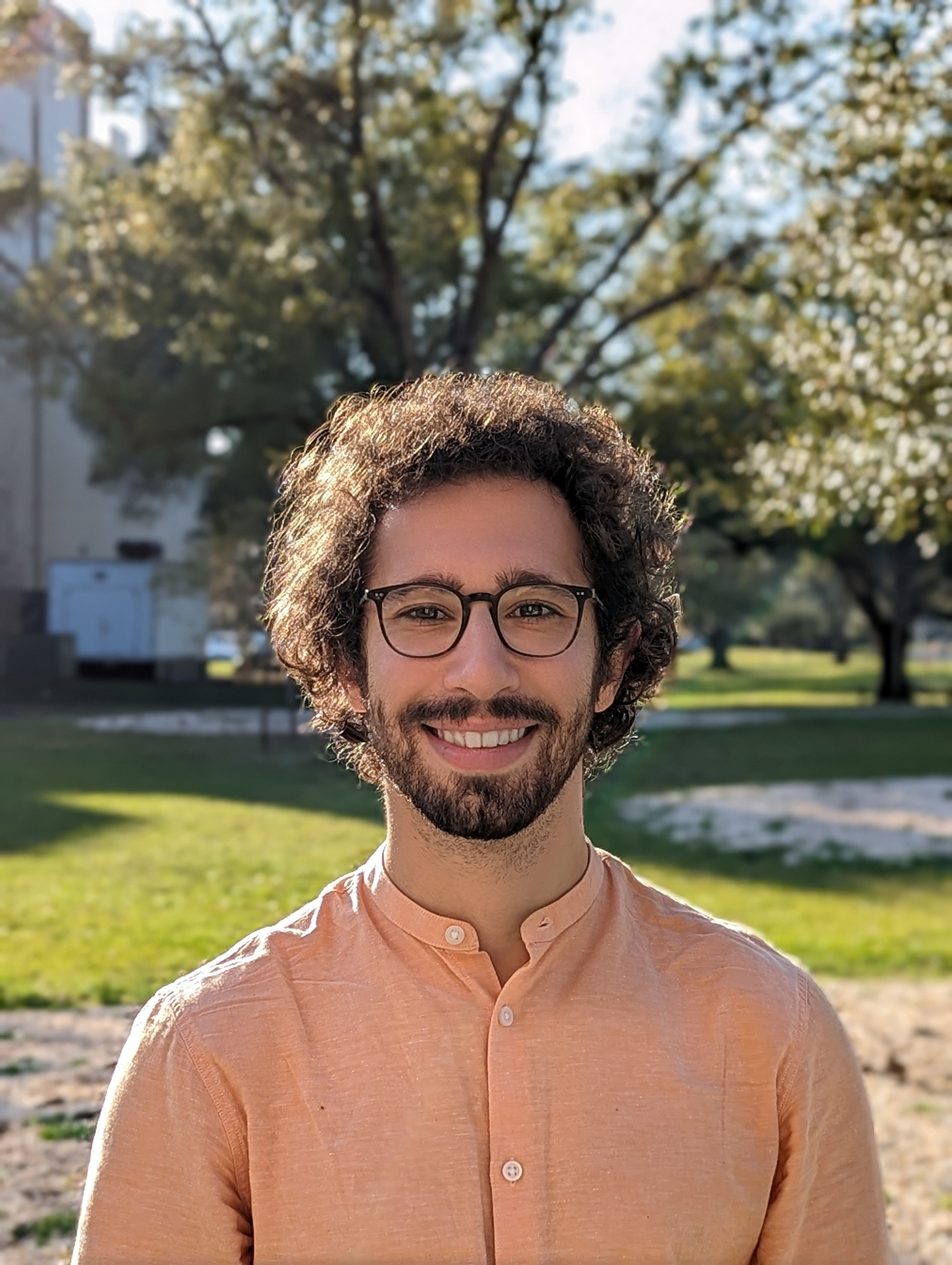## Postdoctoral Fellow at University of South Florida### Vincenzo   Pallozzi Lavorante

I am currently a post doc fellow at the University of South Florida, working under the supervision of Prof. Micheli. I am collaborating with Prof. Giulietti at the University of Perugia and Prof. Korchmaros at the University of Basilicata. I am currently a member of the Center for Cryptographic Research at USF.

# Research

### Interests

My research ranges from the study of Galois theory to combinatorial structures, with particular attentions to coding theory, cryptography and permutation polynomials. I also like to explore anything I find attractive and I am keen to enter new areas whenever they comes with some interesting applications.

# Selected Papers### "Optimal locally recoverable codes with hierarchy from nested F-adic expansions".

#### A. Dukes, G. Micheli and VPL

In this paper we construct new optimal hierarchical locally recoverable codes. Our construction is based on a combination of the ideas of ,  with an algebraic number theoretical approach that allows to give a finer tuning of the minimum distance of the intermediate code (allowing larger dimension of the final code), and to remove restrictions on the arithmetic properties of q compared with the size of the locality sets in the hierarchy. In turn, we manage to obtain codes with a wider set of parameters both for the size q of the base field, and for the hierarchy size, while keeping the optimality of the codes we construct.### "A general construction of permutation polynomials of Fq2."

#### X-d. Hou and VPL

Let $r$ be a positive integer, $h(X)\in\Bbb F_{q^2}[X]$, and $\mu_{q+1}$ be the subgroup of order $q+1$ of $\Bbb F_{q^2}^*$. It is well known that $X^rh(X^{q-1})$ permutes $\Bbb F_{q^2}$ if and only if $\text{gcd}(r,q-1)=1$ and $X^rh(X)^{q-1}$ permutes $\mu_{q+1}$. There are many ad hoc constructions of permutation polynomials of $\Bbb F_{q^2}$ of this type such that $h(X)^{q-1}$ induces monomial functions on the cosets of a subgroup of $\mu_{q+1}$. We give a general construction that can generate, through an algorithm, {\em all} permutation polynomials of $\Bbb F_{q^2}$ with this property, including many which are not known previously. The construction is illustrated explicitly for permutation binomials and trinomials.

# Contact Details

12010 USF Cherry Dr, Tampa, FL 33620

Office: CMC 028

vincenzop@usf.edu